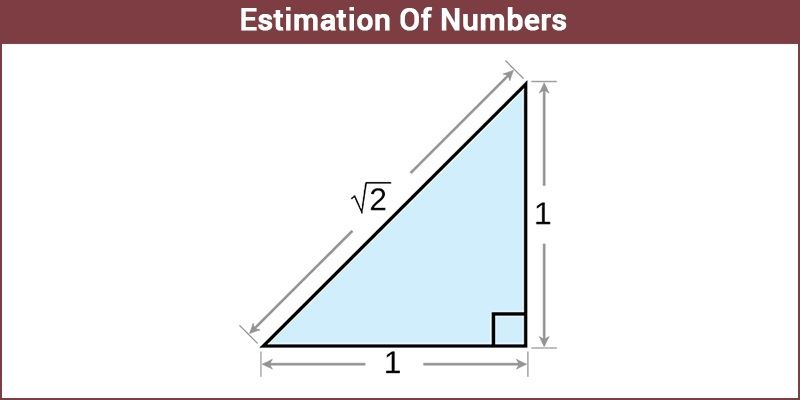# Estimation Of Numbers

Estimation of Numbers is an integral aspect of mathematics, and we engage in it every day. Be it estimating the bill, a person’s weight or solving a Maths problem. But of course, approximate is not good enough sometimes, often we need to know the exact number.

We involuntarily participate in estimation all our lives. Be it a kid estimating how much chocolate he can get out of his parents or a stock market analyst estimating the trends in the markets. Just the scale varies. If one had to define estimation, it would sound something like this, estimation is to form an approximate judgment or opinion regarding the worth, amount, size, weight, etc. or in other words calculate approximately.## Estimation of Numbers by Rounding Off Method

When it comes to mathematics, before we even get down to solving the problems, we tend to estimate its answers in our heads. Although we prefer the exact answer in Maths, still sometimes we tend to approximate the answers to represent them in a simple way. Rounding off is a kind of estimation used quite often in mathematics.

If the digit on the right side of the decimal (.) is less than 5, then we round off the number as per the digit present on the left side of the decimal. But if the digit on the right side of the decimal is greater than 5, then we round off the number as per the digit present on the right side of the symbol.

Take an example like 5.3, since 3 is lesser than 5 the number will be rounded off to 5. While on the other hand if the number is 5.6, then it will be rounded up to 6 since 6 is greater than 5. Hence, we can write:

5.3≈5

5.6≈6

where the symbol (≈) shows the approximation between the two values.

Sometimes, on a large scale, we also approximate the whole numbers to calculate or estimate a value. For example, take 456; it would be 460, while 234 will end up as 230. Again here you will see, based on the last digit of the whole number, the approximate value is taken.

### Uses of Estimation of Numbers

Estimation of numbers is the process of estimating/approximating or rounding off the numbers in which the value is used for some other purpose in order to avoid complicated calculations. There is a difference between the terms exact and estimation.

In mathematics, the term ‘exact’ refers to ‘equal’ and the ‘estimation’ refers to the ‘approximated’ value. Estimation of numbers saves time. After estimating the numbers, we don’t require calculators to solve the problem. But in mathematics, we always require an exact answer. Estimation is the process of finding the number which is close enough to the exact solution. But it is not an exact answer.

In real life, for instance, there were around 500 people present in the marriage hall. Hence, it is an approximate figure and the actual number.

## Video Lesson on NumbersTo learn more Maths-related concepts, stay tuned with BYJU’S – The Learning App and explore more videos.

Test your knowledge on Estimation Of Numbers